This blog has moved ! New look and new articles on www.naturelovesmath.com/en/

# About numbers, episode 4 : infinite numbers

 Medium : for amateurs« Eternity is really long, especially near the end. »
Woody Allen (1935 - )The lemniscate, symbol of infinity Source favim.com
What is infinity ? How to grasp such a concept, by definition so immense that nothing can contain it ?
As surprising as it may seem, the answer lies not in philosophy, but really in mathematics.
We will see that it is possible to define infinity in a rigorous and coherent manner.
There even exists numerous different infinities : precisely an infinity of them !
Three separate notions of infinity play a major role in mathematics :
The ordinal infinity, defined as a number greater than any natural number, the cardinal infinity as the number of elements of an infinite set, and the infinity used in calculus and especially limits as an unreachable element.

However, the latter of the three is misleading, being more representative of the concept of unboundedness than that of infinity, as was explained to us by Riemann and Einstein.
Of course it doesn't stop there, and the mathematicians' imagination can give us a lot more definitions of infinity, which shows us how limitless the human mind can be.

## To infinity and beyond : the transfinite ordinal numbers

We saw in the first episode of this series about numbers that they can be defined in two different ways. The concept of ordinal numbers is tightly linked to that of order. Ordinal numbers "follow each others" and it is only their order that defines them. After the number 0 comes the number 1, then 2, and so on.

Now to extend the order of natural numbers to infinity, a problem arises : which number does it follow ? The answer is all. Infinity follows all of them.

To get this, we need to forget the concept of number and only use the fundamental objects we use to define them : sets. In the same way that $0$ represents the empty set $\{\}$, the number $1$ the set that contains it, i.e. $\{\{\}\}$, the $2$ containing the both of them $\{\{\},\{\{\}\}\}$, etc. Each set contains its predecessors. The first infinite ordinal will contain all of the ordinal natural numbers.

The least infinite ordinal is called $\omega$ and is defined as follow : $\omega=\{\{\},\{\{\}\},\{\{\},\{\{\}\}\},\{\{\},\{\{\}\},\{\{\},\{\{\}\}\}\},\dots\}$.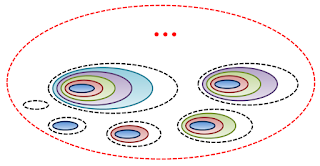Visual representation of the first transfinite ordinal $\omega$, set of all finite ordinals. Of course it's impossible to draw them all, hence the suspension point.

Naturally, the construction can be iterated... to infinity ! We then obtain an infinity of transfinite ordinals :
The next one is written $\omega+1$ and verifies $\omega+1=\{\{\},\{\{\}\},\{\{\},\{\{\}\}\},\{\{\},\{\{\}\},\{\{\},\{\{\}\}\}\},\dots,\omega\}$
Then $\omega+2=\{\{\},\{\{\}\},\{\{\},\{\{\}\}\},\{\{\},\{\{\}\},\{\{\},\{\{\}\}\}\},\dots,\omega,\omega+1\}$
etc.

That's a lot of infinites, don't you think ? Well you've seen nothing, as we barely scratched the surface. It is possible to continue, by defining the set of all the preceding ordinals :
Then we have the transfinite $\omega\cdot 2$ also equal to $\omega+\omega$. It contains all of its predecessors $\omega\cdot 2=\{\{\},\{\{\}\},\{\{\},\{\{\}\}\},\{\{\},\{\{\}\},\{\{\},\{\{\}\}\}\},\dots,\omega,\omega+1,\omega+2,\dots\}$
Then comes $\omega\cdot 3=\{\{\},\{\{\}\},\{\{\},\{\{\}\}\},\{\{\},\{\{\}\},\{\{\},\{\{\}\}\}\},\dots,\omega,\omega+1,\omega+2,\dots,\omega\cdot 2\}$
etc.
We can go on indefinitely, and obtain $\omega^2, \omega^3, \dots, \omega^{\omega}, \dots, \omega^{\omega^{\omega}}, \dots,$ etc.

Staggering, isn't it ?

In a more visual fashion :

## Counting the infinity : transfinite cardinal numbers

We saw that natural ordinal and cardinal numbers are equal. Each finite cardinal was defined as the number of elements in the set defining the corresponding ordinal. Well, more acurrately, cardinal numbers are defined, again using the concept of equipotence (sets in bijection, or "one to one correspondance") like so :

Definition : The cardinal of a set is the least ordinal number with witch it is equipotent.

Since the sets that define natural ordinal and cardinal numbers are equipotent, we have indeed equality between them. But what about infinite sets ?

The smallest infinite cardinal, denoted by $\aleph_0$, is the number of element in the set of all natural numbers $\{0,1,2,3,4,\dots\}$ and if we replace each number by its definition in terms of sets, it is the number or elements of $\{\{\},\{\{\}\},\{\{\},\{\{\}\}\},\{\{\},\{\{\}\},\{\{\},\{\{\}\}\}\},\dots\}$, so $\omega$.
So we have $\aleph_0=\omega$.
Beware : $\aleph_0$ equals $\omega$, but its properties are different, not being tied to any order, but to the concept of cardinality, as pictured in this image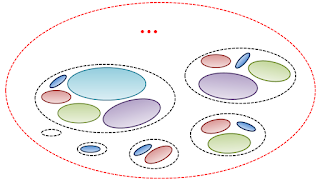Visual representation of the first infinite cardinal $\aleph_0$, more or less an "unordered $\omega$".

But for the next ones, it becomes a bit complicated. The following transfinite cardinal, denoted by $\aleph_1$, is not $\omega+1$. Why ? Because $\omega$ and $\omega+1$ are equipotent. They have the same number of elements. Impossible, you would think ! Since $\omega+1$ contains one more element than $\omega$ ! Yes, it is true, but to add an element to an infinite cardinal does not in fact change its quantity, it stays the same cardinal number... It is Hilbert's paradox of the Grand Hotel.

### Hilbert's hotel, or the part that equals the whole

This thought experiment is attributed to the mathematician David Hilbert (1862 - 1943). Imagine a hotel with infinitely many rooms. Let's all the rooms be occupied. Suppose a new guest arrives at the hotel and asks for accomodation. Even if the hotel is full, it is possible to make room for the new guest !The infinite hotel of Hilbert is full

To that end, we just have to ask each client to change room and to take the next one : since the hotel is infinite, everyone will find a room and the first one will be available for the newcomer...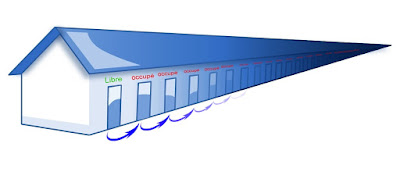A room is available

Of course, it is possible to reiterate the experiment as many times as we want. And what's more, even with an infinity of new guests, we can make room for everyone ! We just have to ask each occupant to leave his $n$th room to go to the $2n$th one. Then every even-numbered room will be occupied, and the odd-numbered ones will be free and ready for our boatload of new guests.An infinity of rooms made available !

The thing is, it has to be a countable infinity for it to work, that is to say equal to the number of natural numbers, integers or rational numbers (see the preceding episode about rational numbers for more about countability).

So the arithmetics of infinite numbers is very peculiar. If one adds $1$ to an infinite ordinal that gives us another ordinal number, $\omega+1$ being strictly greater than $\omega$, but adding $1$ to an infinite cardinal doesn't change it : $\aleph_0+1=\aleph_0$.

As a consequence, for each natural number $n$ we have $\aleph_0+n=\aleph_0$, and even $\aleph_0+\aleph_0=\aleph_0\times\aleph_0=\aleph_0^{n}=\aleph_0$.
These strange arithmetic rules are true for any transfinite cardinal.

So in plain english : we can indefinitely add to an infinite ordinal without changing its cardinal, that's why the next cardinal will be a far greater ordinal than its successor.

We define $\aleph_1$ as the cardinal of all countable ordinals, also denoted $\omega_1$. Note that this set is the smallest uncountable set, and that it is not pictured in the preceding representation of transfinite ordinals, because it is too big.

$\aleph_0$ is the cardinal of $\mathbb N$ the set of all natural numbers, but also that of $\mathbb Z, \mathbb Q$ and in fact all of the infinite countable sets.
The cardinal of the set of real numbers is $2^{\aleph_0}$. It is an uncountable infinite.
Do we have $\aleph_1=2^{\aleph_0}$ ? It's the continuum hypothesis, and the answer is... yes and no. Yes since the hypothesis does not contradict set theory, and no because its negation doesn't either ! This proposition is a well known example of undecidable statement.

## The secret nobody told you : infinity is not the absence of limit !

Most dictionaries and encyclopedies define infinity as the absence of limit or bound.
Unfortunately, that is not correct. This definition better fits the term "unboundedness", which is a completely different mathematical concept.
According to Bernard Riemann :

we must distinguish between unboundedness and infinite extent, the former belongs to the extent relations, the latter to the measure-relations.
Bernhard Riemann, On the Hypotheses which lie at the Bases of Geometry, Nature, Vol. VIII. Nos. 183, 184, pp. 14-17, 36, 37, translated in english by William Kingdon Clifford.
Mémoires de la Société royale des sciences de Göttingue, t. XIII ; 1867 Oeuvres de Riemann, 2ème édition, 1854, traduction française J. Houël.

Let's take a geometrical example, easy to visualize : a sphere. Its surface is clearly finite, since it can be entirely filled with smaller surfaces of a fixed width : we can calculate its area, given by the well known formula $4\pi \times R^2$.
But this surface is also unlimited : we know for a long time that the earth has no edge, and that we could travel on it indefinitely without ever meeting any limit to our movements.
Not convinced by this example ? Don't trust me, but you can trust the person who gave it first, Albert Einstein who described it brilliantly in its article "Geometry and experience", lecture before the Prussian Academy of Sciences, January 27, 1921, reprint by Springer, Berlin, 1921.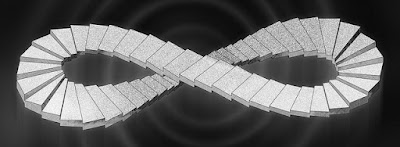Unbounded finite stairs Source Turb(l)o(g), original credit Mathieu Nauleau

But then, what is the infinity of analysis if not the unboundedness ?

## The infinity in analysis

First, a little bit of history.
To the layman, infinity is linked to one symbol : the lemniscate $\infty$. Indeed, it is the first symbol representing infinity that has been universally used. It was introduced by the english mathematician John Wallis (1616 - 1703) in 1655 (In its "De sectionibus conicis", Pars I, Prop.1, and Opera mathematica, vol 1, p297, Oxford, 1695).
Its most common use was popularized by K. Weierstrass (1815 - 1897) in his functions theory. It meant both an actual infinite when used without sign, and a limit either denoted $+\infty$ or $-\infty$.

Georg Cantor (1845 - 1918) who invented the transfinite numbers, used it before replacing it with $\omega$, because the symbol $\infty$ was already used in the literature for the undetermined infinite quantities. (Florian Cajori, History of mathematical notations, vol II, Cossimo New-York, 2007, p42).John Wallis Source MacTutor History of Mathematics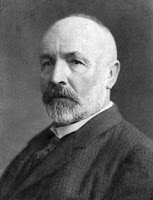Georg Cantor Source Wikipedia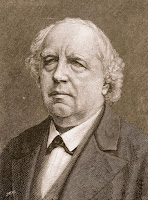Karl Weierstrass Unknown source

We will now see the exact signification of the lemniscate, in different contexts (sometimes a little bit complicated, so hang on if you're not into math, and sorry for the oversimplification if you are). The important thing to remember is that $\infty$ generally is not a number !
One of the most difficult thing to do in mathematics is to get rid of all preconceived notions surrounding a symbol, and give it different significations depending upon the context. This symbol of infinity is a perfect example.

### The indeterminate infinite of analysis and limits

In analysis, there are three different uses of infinity :

1. A practical notation
When infinity is used as a bound of a sum, a product or an integral defined over $\mathbb R$ or $\mathbb N$ like for example $\displaystyle\int_{-\infty}^{+\infty}f(t)dt$ or $\displaystyle\sum_{n=0}^{\infty}f(n)$, it only means over the set of all real numbers or natural numbers. We note $\mathbb R=]-\infty, +\infty[$ and we added nothing to $\mathbb R$ since the square brackets are positioned so that infinity is not comprised in the set. It is only a useful way to designate all of the real numbers, and that the operation is applied onto all of them. Of course, it implies that such a manipulation over an infinity of elements is possible and in a way it necessitates the concept of actual infinity, but it doesn't change anything. Here we are not talking about infinite numbers, but only about notation. Probably an improper use of it since it implies that $\mathbb R$ is included in $[-\infty, +\infty]$ which is not yet defined in this context, even if it doesn't change anything in the calculations.

2. In the extended real number line $\overline{\mathbb R}=\mathbb R\bigcup\{-\infty,+\infty\}$, the infinities are indeed numbers (not real numbers though), but they are indeterminate infinities : these infinities are neither $\omega$, nor $\aleph$, they "represent them all" in a way. But be careful, it does not mean that $+\infty$ is some kind of super infinity greater than them all in the sense of set theory, because there does not exist any set of all ordinals or set of all cardinals, in the same way that there does not exist any set of all sets. Their existence would be contradictory, so they are more "universal sets" with different properties than mere sets, and it's a whole other story. So why are these infinite numbers different than  transfinite ones ?

When infinity is the result of a calculation like $\displaystyle\sum_{n=0}^{\infty}n=+\infty$, it is natural to think of it as a number since we only added numbers, but it generally is not so in analysis. It merely points to the concept of divergence, as opposed to that of convergence. If we add an infinity of numbers and get a finite result, we say the series converges. In the other case, it diverges. So saying that the sum equals infinity is not per se an improper use of the term, but an imprecise one : is it a countable infinity ? uncountable ? If we add all the natural numbers, integers or rational numbers, the results would be countable (the union of countable sets is countable thanks to the axiom of choice), but which one since we've seen there are an infinity of countable infinities ? If we sum over an interval of real numbers, in case of divergence we would obtain an incountable infinity, so a different one. But we denote it $+\infty$ in both cases ! Simply because the distinction is an unnecessary complication in the divergence context.

Now what happens if we need to make some calculations involving both infinites ? Well, since we do not know "to which degree they are infinite" in a manner of speaking, in most cases we cannot say what the result is, which is why it is called an indeterminate form. $+\infty -\infty$, $\dfrac{\infty}{\infty}$ are indeterminate, just as the division by zero. But $0\times\infty$ is well defined and equals $0$, since multiplying by zero gives the same result for ordinal numbers than cardinal ones, finite or not.

3. A priori, calculations with limits, since they are done on the extended real number line, should obey the same rules, shouldn't they ? Absolutely not ! The elements of the extended real number line are used, but in a very different context :
A limit is not the result value of a function, and it isn't even necessarily a number !
For example, a function can approach a value continuously and always be greater, or the converse. So the limits can be different according to which "path" the function takes...

So what's a limit ? Beware if you're allergic to math formulas, it's gonna hurt :
To say that $L$ is the limit of a function when $x$ approaches $y$, which is noted $\displaystyle\lim_{x\to y}f(x)=L$, is to say :

$\forall \epsilon>0, \exists \eta>0, \text{ if } |x-y|<\eta \text{ then } |f(x)-L|< \epsilon$

In english, if $x$ approaches $y$ closely enough, then $f(x)$ approaches $L$ as closely as we want. So you see that with such a definition we cannot simply add or multiply limits as we wish... When the limits are finite there's no problem, the limit of a sum of functions is the sum of their limits and the same goes for products. BUT as soon as $0$ and $\infty$ come in, the problems begin ! In the calculations with limits, we tend to use the same notations we would with numbers, but it's only by habit, since we don't really do these operations on the limits, particularly with infinite ones. We do not do transfinite arithmetics, and so $0\times \infty$ for example is not defined, it's an indeterminate form. It's not a product of numbers, but a product of limits, which we have to read like so : the limit of the product of a function approching $0$ by a function approaching $\infty$ is indeterminate. It is neither the product, nor the sum, nor anything else, there is no know method to get the resulting limit by using only the given limits, we need more information about the functions. In some cases it will be $0$, sometimes $\infty$, sometimes even a finite number. It all depends on the functions.
So even if we can see $+\infty$ as a transfinite number, in the context of limits it cannot be manipulated in the same way as the numbers, but as some properties of functions. What we represent by these same symbols are different concepts. Even if it is possible to add all the transfinite numbers to $\mathbb R$ and work up a new theory of limits, we would exit the standard analysis theory and be working in a very different theory...

### The point at infinity, or ideal point

When infinity is used as a value - for a function $f(\infty)=\infty$ for example -, we just added an element to the domain of the function, to "complete it". In the case in which we complete the set of real numbers, we then have the set $\mathbb R\bigcup\{\infty\}$, and depending on the structure we give that set, it obeys different rules :

• If we add a topological structure, we obtain what is called an Alexandrov extension. It is then a topological space equivalent to a circle, we "closed" the real line. We still cannot call its elements numbers, but only "elements", "open sets" and "sets". The functions defined on this domain are not real functions. Typically we have a projective space, in which $\infty$ is just an element, and $f(\infty)=\infty$ only tells us that this element is fixed by the function $f$. No numbers are present here.

• If we give it a group structure, that is to say more or less that we define an operation on it, say $+$, we are still not adding numbers ! It gets complicated - and that is not our goal here - but we can resume the situation by describing those elements as linear transformations "quotiented" by homothetic tranformations (quotiented in a group theory sense, meaning with an equivalence relation)... they are projective transformations in fact. So still not numbers.

• We can add another operation, say a product, but we will still be in a projective space, very far from an algebraic field, and the operations will not be the same as those with numbers...
To sum up those three examples, we completely change the structure of the set of real numbers by adding just one element. We can call it the point at infinity, or ideal point, since it is more of a geometrical object than a number. The set $\mathbb R\bigcup\{\infty\}$ is called the projective extended real number system.The point at infinity of a projective space Source Images des mathématiques

## So what the heck is infinity ?

To conclude, let's resume : there are numerous concepts of infinity, all with their proper rules, all perfectly coherent. Infinity is not the same as inboundedness and some infinites are numbers, but some are not.
They are objects that escape our representation : impossible to see them in the real world, and even impossible to see them in our imagination, the infinities represent elements or sets that go way beyond the capability of our thoughts. After all, our brains are made of a finite number of neurons, cells, molecules or atoms...
Sure, they are unsettling, paradoxical even, sometimes undecidable. But it only means we're not familiar with them, and we need to make a considerable amount of effort to grasp them and manipulate them. Finally, they are the roots of a fascinating universe, which immensity goes infinitely beyond our wildest dreams !

### Other posts on the topic «About numbers»

#### Episode 3 : rational numbers

Crédits :
Buzz Lightyear : renders-graphiques.fr

$2\infty$&$<$ Tee-shirt : tshirtroundup.com# Comments

9 Responses to "About numbers, episode 4 : infinite numbers"

Yoda of Borg said... August 24, 2012 at 7:10 AM

Your image ordinaux+transfinis.png with the ordinals spiraling into the center is labeled incorrectly. It is correct up to w.2, but the next one should be w.2+1 not w.3. w.3 is a limit ordinal, but the line you've put it next to is a successor. Where you have w^2 is where w.3 should be, where you have w^w is where w.4 should be. Starting at the top, each inward loop represents a higher power of w, so that after the second loop, near your last red "..." is where w^2 should be. This image does not illustrate every ordinal, the center of the image, where the representation ends is w^w.

Yoda of Borg said... August 24, 2012 at 7:26 AM

Another comment about ordinaux+transfinis.png: I don't mean to nitpick, but while you're fixing that image, the venn-diagram-like ovals for w+2 and w+3 are wrong. The one for w+1 is union(w,{w}) = {w,{w}} which is right, but the one for w+2 visually represents union(w,{w},{w}) which has {w} repeated, so it is union(w,{w},{w}) = union(w,{w}) = {w,{w}} = w+1. It should be w+2 = union(w+1,{w+1}) = {w+1,{w+1}} = {{w,{w}},{{w,{w}}}}. Similarly for w+3, you display union(w,{w},{w},{w}).

Johann said... August 24, 2012 at 12:56 PM

You're absolutely right I skipped a few of them in the spiral !
(a few is quite an understatement when talking about transfinite numbers^^).
I follow you right until w^2, but then I'm not sure any more. You say "each inward loop represents a higher power of w", but in the spiral the inward loop after w^2 becomes half a loop, then a quarter, and an eighth of a loop, etc. so in my opinion w^w is right under w^2.

And yes the last diagrams in the picture are approximate because the elements are becoming smaller than pixels so I didn't think anyone would see a difference between {w,w} and {w,w+1}... I corrected both. Can you tell ? ^^ BTW w+2={w+1,w,1,2,3,4,...}, you forgot the natural numbers in your last comment ;-)

Thanks a lot !

Yoda of Borg said... August 25, 2012 at 8:50 AM

Just a few. ;-)

The image is much better (but needs another update).

You're right that the circles get so small they're hard to read. I can still read w+2 though, and it's correct. w+3 is too small to read clearly (so it's a good place to stop), but before it was clear enough to read and was clearly incorrect. While I can't read it, I'm sure you updated it correctly and it won't mislead now. :-)

Now that I look at it, your oval diagrams for 2, 3, and 4 aren't right either. You have 2={1}, 3={2}, 4={3}. 2 should have a blue oval in it, and a red oval with a blue oval inside it. After fixing that, the oval notation will probably be more illuminating when people see the set 2 has 2 ovals in it, 3 has 3 ovals in it, etc.

When I first saw this spiral representation, it took me quite some time before I was sure I was reading it correctly. The think to keep in mind is that the entire spiral is not sophisticated enough to indicate every ordinal or even every countable ordinal. The vanishing point in the center of the spiral is w^w. You've got the first 2 loops correct now. Technically w^0=1 so the one-power-of-w-per-loop rule would only be literal for the outside loop if 1 (instead of 0) were directly above w.

Since your red labels are correct now to w^2, let's start there at the end of the 2nd loop. The next line after w^2 is its successor w^2+1, then w^2+2 ... so that the next limit 1/4th of the way around the 3rd loop is the limit of all those successors: w^2+w. This is followed by it's successor w^2+w+1 and then w^2+w+2... with the next limit being w^2+w.2. This pattern continues for the 2nd quarter of the 3rd loop so that the limits w^2, w^2+w, w^2+w.2, w^2+w.3 limit at the bottom of the third loop with w^2+w.w = w^2+w^2 = (w^2).2 (where you have w^3 instead). The same continues so that where you have w^4 is actually (w^2).3, w^5 is actually (w^2).4 etc so that the limit of these limits at the top/end of the 3rd loop (where you have w^w) is (w^2).w = w^3.

Loop 4: The top starts with w^3 at the end of loop 3. At the right of the loop after the first 1/8th is w^3+w. The 2nd 1/8th contains w^3+w, w^3+w.2, w^3+w.3, ... limiting to w^3+w^2 after the first 1/4th at the right of the loop. At this point the pixelation stops us from figuring out what's going on by looking directly at the hash marks, but we have enough info from the outside loops to extrapolate a general pattern. The first half of each loop has as much complexity as EVERYTHING that came before that current loop, so it is the value at the top/beginning of the loop times 2. (With the exception of the outside loop. For it to follow the pattern of the rest of the diagram, 1 should be at the top of the outside loop, 2 would be at the bottom, 3 would be 3/4 of the way around the loop, 4 would be 7/8th of the way around etc. But this would give a very sparse looking pattern for the finite ordinals and indeed each limit of successors would look sparse/hard to read, and so the pattern within a set of successors leading up to their limit is different than the pattern between limits. If the spacing between successors is geometric, it's a very gradual on, but it's clear that the spacing between the limits is geometric.) Just like the complexity of everything in the entire spiral before the current loop is duplicated in the first 1/2 of the current loop, it's all duplicated again in the next 1/4th, and again in the next 1/8th, and again... so that the value (alpha) at the start of a loop is doubled after the first 1/2 of the loop: (alpha).3, tripled after the next 1/4th: (alpha).3, quadrupled after the next 1/8th: (alpha).4), ... so that the limit of each loop is (alpha).w or one power of w higher than the start of the loop. This is why loop 1 ends with w^1, loop 2 ends with w^2, loop 3 ends with w^3, ... and since we have w loops, the limit of this representation, the point at the very center of the image where the spiral vanishes, is w^w. (Hope that helps.)

Yoda of Borg said... August 25, 2012 at 9:18 AM

I'm sorry. I didn't address you last sentence in the comment I just published.

You are correct that w+2={w+1,w, 0 <-- ;-), 1,2,3...}. It seems that in trying to illustrate the nested nature of your ovals, I abused the notation. It is still correct that w+2=union(w+1,{w+1}), but when I tried to show the nested result representing that union I didn't have a way to say "the elements INSIDE set X". Thus when I said {w+1,{w+1}} I meant take the elements INSIDE w+1 and put them in a set with the element INSIDE {w+1}. So my statements were correct when I phrased them in terms of a union, but ALL of my nested brackets and w's are wrong. Each ordinal needs it's (internal) brackets peeled off before being put in the visible brackets to make a correct statement.

Johann said... August 25, 2012 at 2:07 PM

About my oval diagrams :
They don't intend to represent the Von Neumann definition of ordinals, i.e. the sets I used to define them here. Only the fact that they are ordered.

You'd be right if they were representing numbers without the ordinal/cardinal distinction. Because cardinal are also by definition ordinals (the same for finite ones, not all the same for infinite ones), the complete representation you want to use wouldn't show any difference between the two.

Think of the diagrams as models : they are not exact graphic representations (like functions' graphs or geometrical objects in some predefined space), but objects that bare a resemblance, that have a common property. In a model, like in physics, we have to overlook the differences to focus on the common properties (like when physicists say "let's assume earth is a perfect sphere...").

So when you say you "abused the notation" in your last comment, you didn't, I think you just assumed it was intended as an exact representation, but it's just a model.

Nested ovals represent the order of ordinals, mixed up ovals represent the cardinality (technically cardinals are ordered too, but I want to hide it in that model), and mixed-up nested ovals would represent numbers with the two properties.

Maybe it's not the best way to represent them but I find very illuminating the distinction between ordinals and cardinals (it's a nice duality, we can easily think of ordinals without cardinality but often forget that we can also think of cardinals without the order), and that's only what the diagrams are here for.

Thanks again for your input in the spiral, you seem right again, and I'll add the corrections soon. Your explanation was enlightening, I never put too much thought into it, just figured it was a nice way to picture them^^.

Johann said... August 25, 2012 at 2:32 PM

Also, I forgot to add that for the transfinite ordinals, I could not nest all the ovals in my diagrams like I did with the finite ordinals, otherwise it would have been way too messy and unreadable, so for the sake of clarity it's a bit more imperfect.

Yoda of Borg said... August 25, 2012 at 8:18 PM

Ah. I've only ever had a course on Von Neumann set theory, so cardinals without order is unfamiliar to me. (We never actually stated that we were working in Von Neumann set theory, but afterwards when I learned about other models of set theory it was very similar and I imagine the difference was just a matter of presentation.)

It occurs to me that now that we've changed things, anyone who comes along later may wonder what we're going on about, but hopefully they'll find our discussion illuminating.

Johann said... August 26, 2012 at 2:49 PM

I like to see cardinality in an invariant-theoretic kind of way : it's THE invariant under bijections between sets.

Anyway, glad for this discussion.

### Post a Comment

You can use HTML tags b, i, a.

# Nature loves math...

You love math ? Then come and see some of its beautiful use in physics. You hate math ? Pass over the complicated formulas ! Your imagination is all you need to see the beauty in it...